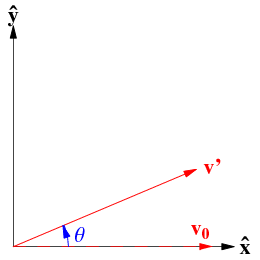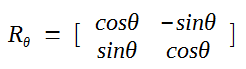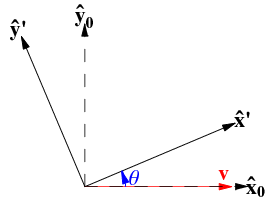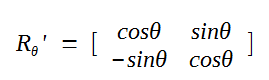# Rotation Matrix

When discussing a rotation, there are two possible conventions:

• rotation of the object relative to fixed axes
• rotation of the axes

#### 1. Rotation of the object relative to fixed axes

In R2 space, consider the matrix that rotates a given vector v0 by a counterclockwise angle θ in a fixed coordinate system as shown below.Then the rotation matrix is:So

v' = Rθ v0

#### 2.  Rotation of the Axes

On the other hand, consider the matrix that rotates the coordinate system through a counterclockwise angle θ.The coordinates of the fixed vector v in the rotated coordinate system are now given by a rotation matrix which is the transpose of the fixed-axis matrix.

As can be seen in the above diagram, this is equivalent to rotating the vector by a counterclockwise angle of -θ relative to a fixed set of axes.

So the rotation matrix is:This is the convention commonly used in textbooks such as Arfken (1985, p. 195).